In :
import pyfits

This spacecraft file covers the period from August 5, 2008 (start of science operations) to JUne 3, 2013. We can use this to explore the observations made over the course of the mission.
In :
sc_hdulist = pyfits.open('L1306030907575650A47F32_SC00.fits')

In :
sc_data = sc_hdulist.data

In :
%pylab inline

Welcome to pylab, a matplotlib-based Python environment [backend: module://IPython.zmq.pylab.backend_inline].

In :
figsize(16,8)
dates = epoch2num(978307200+sc_data.field('START'))
plot_date(dates,sc_data.field('LAT_MODE'),'.')
ylim(1.1,5.1)
show()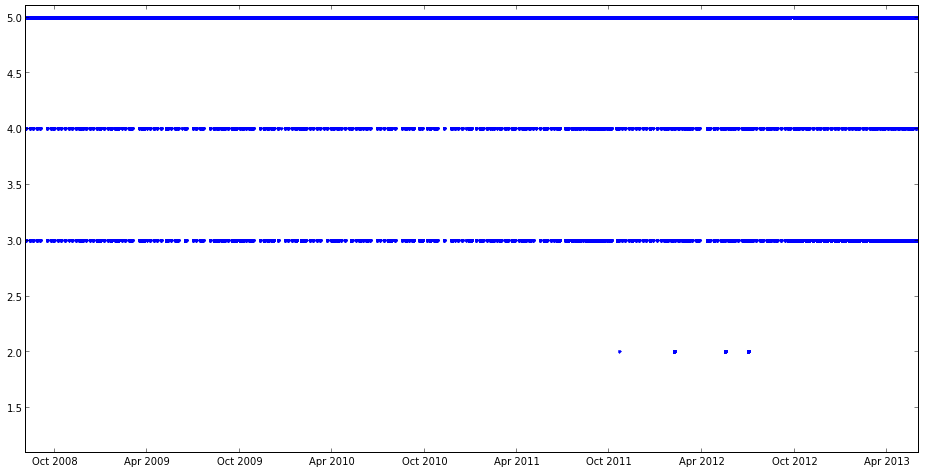This is a plot of LAT_MODE vs time. 5 is survey mode, 4 is maneuver mode (slewing to or from a target), 3 is pointed mode and 2 is sunpoint. Each week we spend a mixture of time in survey, maneuver and pointed modes. To get a sense of the fraction of time that we spend in each mode, we need to look at a histogram.
In :
sum(sc_data.field('LAT_MODE')>0)

Out:
4279194
In :
figure()
w0=np.ones_like(sc_data.field('LAT_MODE'))*1.0/4279194
n,bins,rectangles = hist(sc_data.field('LAT_MODE'),weights=w0
)
#yscale('log')
show()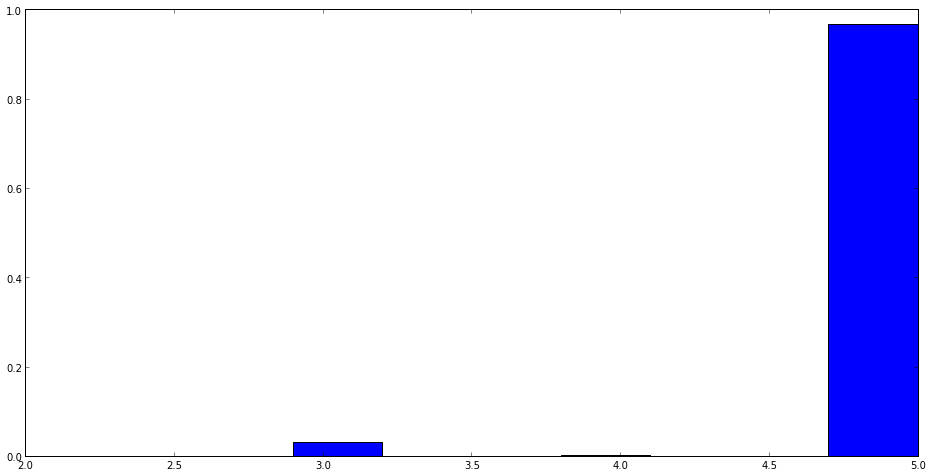The height of each column is the fraction of the mission that we have been in that mode. We can get this directly from the histogram quantities. More than 96% of the time is in survey mode, 3% in pointed mode and the remainder in maneuver and sunpoint.
In :
float(sum(sc_data.field('LAT_MODE') == 5)) / float(sum(sc_data.field('LAT_MODE') > 0)) # fraction survey mode

Out:
0.9667035427699703
In :
float(sum(sc_data.field('LAT_MODE') == 4)) / float(sum(sc_data.field('LAT_MODE') > 0)) # fraction maneuver mode

Out:
0.0024315326671331097
In :
float(sum(sc_data.field('LAT_MODE') == 3)) / float(sum(sc_data.field('LAT_MODE') > 0)) # fraction pointed mode

Out:
0.030813513012029836
In :
float(sum(sc_data.field('LAT_MODE') == 2)) / float(sum(sc_data.field('LAT_MODE') > 0)) # fraction sunpoint mode

Out:
5.1411550866822114e-05
It is a little tricky to see details of what is happening for a plot that covers more than 4 years. So let's switch to one that covers just the past year.
In :
sc_hdulist = pyfits.open('L1306031342065650A47F35_SC00.fits')

In :
sc_data = sc_hdulist.data

In :
figure()
dates = epoch2num(978307200+sc_data.field('START'))
plot_date(dates,sc_data.field('LAT_MODE'),'.')
ylim(1.1,5.1)
show()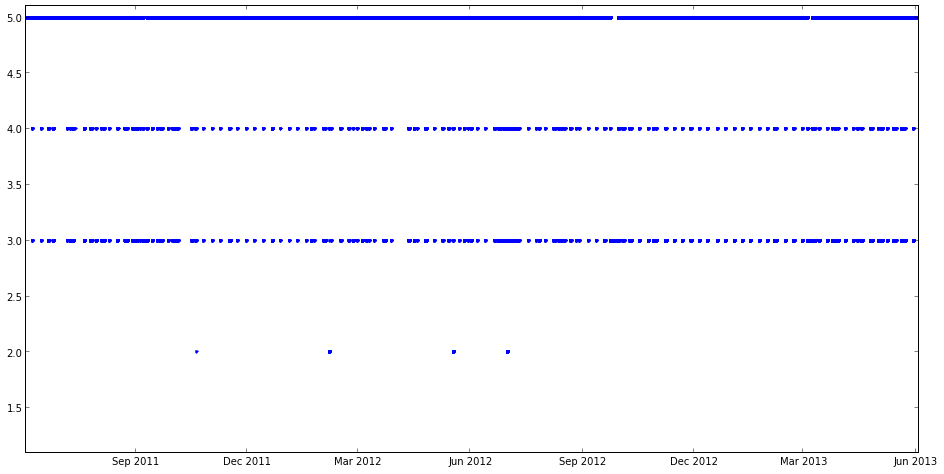In the plot above, we are covering one year of data. We can now see some obvious gaps in survey mode in Sept 2012 and Mar 2013 (there are others, but it is not possible to see them on this scale plot).
In :
figure()
dates = epoch2num(978307200+sc_data.field('START'))
plot_date(dates,sc_data.field('ROCK_ANGLE'),'.')
show()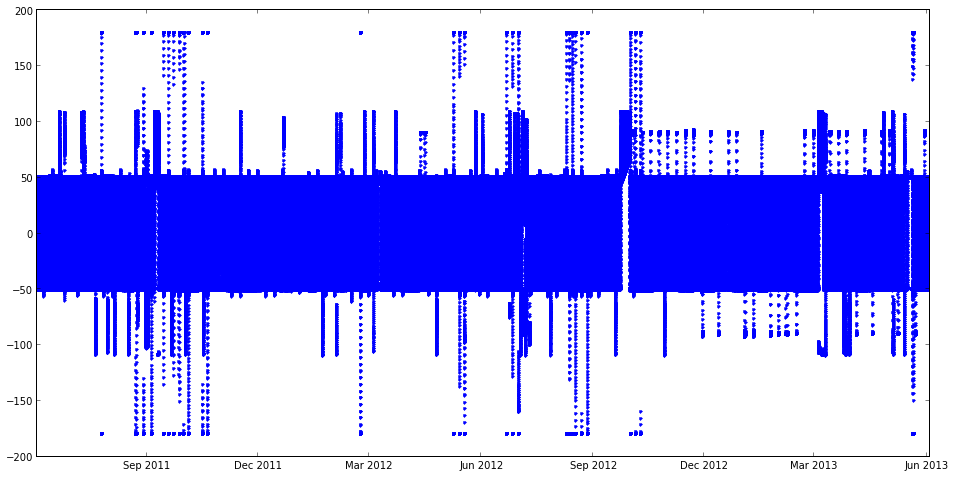This plot shows rocking angle as a function of time. It is now easy to see the periods of non-standard survey mode (any time where were are not solidly between +-50 deg). To find out what these are, you can look at the timeline posted at the Fermi Science Support Center. To look at this, go to observations->observing timeline -> Timeline posting at the Science Suppport Center webpage.
In :
from IPython.display import Image,HTML

In :
HTML("<iframe src='http://fermi.gsfc.nasa.gov/ssc/observations/timeline/posting/ao4/' width='1000' height='500'></iframe>")

Out:
Entry #28 in AO4 is a target of opportunity observation of the Crab Nebula, which was flaring at the time. Let's take a closer look at the week that contains this observation.

An example of a pointed mode observation: Crab Nebula and solar observation in July 2012¶

In :
sc_hdulist = pyfits.open('L1306040628475650A47F60_SC00.fits')

In :
sc_data = sc_hdulist.data

In :
figure()
dates = epoch2num(978307200+sc_data.field('START'))
plot_date(dates,sc_data.field('LAT_MODE'),'.')
ylim(1.1,5.1)
show()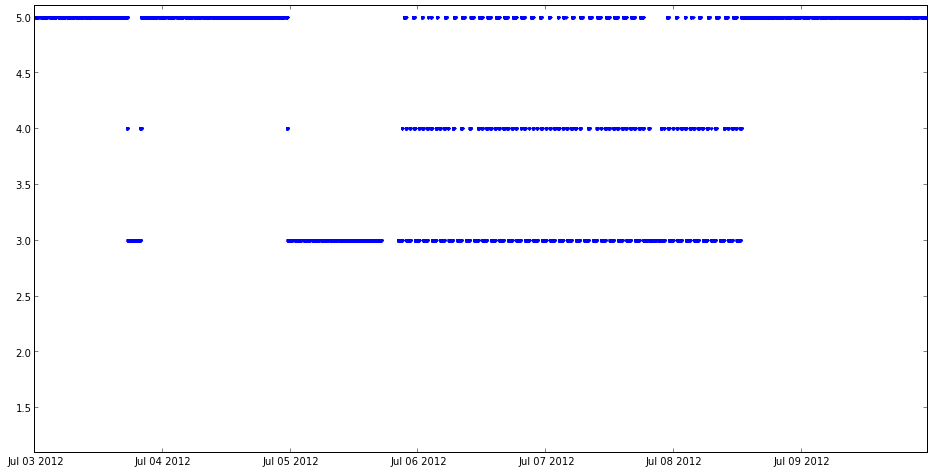At the beginning of the week we had an autonomous repoint for a bright GBM detected GRB120703A. This observation lasted for 2.5 hours. The burst was also detected by Swift, but unfortunately was not seen in the LAT. We received two TOO requests in this week. The first was to look at the Crab nebula, which was flaring and the second was to look at the Sun, which was in a high state of activity. Fortunately, the Sun was close to the Crab, so a pointed observation at the Crab satisfied both requests. The pattern of observations changes. For the first day of the Crab observation, we stayed in pointed TOO mode - i.e. LAT_MODE stayed at 3. During this time, the spacecraft traced the limb of the Earth while the crab was occulted by the Earth. We then replanned the observation to be a mix of pointed observation at the Crab and survey mode. In the next few steps, we'll explore why this made things better. A first step is to take a look at exposure as a function of time for an ROI centered on the Crab. L1306040628475650A47F60_PH00.fits is a photon file for a 10 deg ROI centered on the Crab for the one week period.
In :
lc_data = pyfits.open('L1306040628475650A47F60_PH00.fits')

In :
from gt_apps import maketime, evtbin, filter

In :
filter['evclass'] = 2
filter['infile'] = 'L1306040628475650A47F60_PH00.fits'
filter['outfile'] = 'crab_select.fits'
filter['zmax'] = 100

In :
filter.command()

Out:
'time -p /Users/mcenery/software/ScienceTools/ScienceTools-v9r31p1-fssc-20130410-x86_64-apple-darwin12.2.0/x86_64-apple-darwin12.2.0/bin/gtselect infile=L1306040628475650A47F60_PH00.fits outfile=crab_select.fits ra="INDEF" dec="INDEF" rad="INDEF" tmin="INDEF" tmax="INDEF" emin=100.0 emax=300000.0 zmax=100.0 evclsmin="INDEF" evclsmax="INDEF" evclass=2 convtype=-1 phasemin=0.0 phasemax=1.0 evtable="EVENTS" chatter=2 clobber=yes debug=no gui=no mode="ql"'
In :
filter.run()

time -p /Users/mcenery/software/ScienceTools/ScienceTools-v9r31p1-fssc-20130410-x86_64-apple-darwin12.2.0/x86_64-apple-darwin12.2.0/bin/gtselect infile=L1306040628475650A47F60_PH00.fits outfile=crab_select.fits ra="INDEF" dec="INDEF" rad="INDEF" tmin="INDEF" tmax="INDEF" emin=100.0 emax=300000.0 zmax=100.0 evclsmin="INDEF" evclsmax="INDEF" evclass=2 convtype=-1 phasemin=0.0 phasemax=1.0 evtable="EVENTS" chatter=2 clobber=yes debug=no gui=no mode="ql"
Done.
real 4.14
user 0.17
sys 0.10

In :
maketime['scfile'] = 'L1306040628475650A47F60_SC00.fits'
maketime['filter'] = "(DATA_QUAL==1)&&(LAT_CONFIG==1)"
maketime['roicut'] = 'yes'
maketime['evfile'] = 'crab_select.fits'
maketime['outfile'] = 'crab_mktime.fits'

In :
maketime.command()

Out:
'time -p /Users/mcenery/software/ScienceTools/ScienceTools-v9r31p1-fssc-20130410-x86_64-apple-darwin12.2.0/x86_64-apple-darwin12.2.0/bin/gtmktime scfile=L1306040628475650A47F60_SC00.fits sctable="SC_DATA" filter="(DATA_QUAL==1)&&(LAT_CONFIG==1)" roicut=yes evfile=crab_select.fits evtable="EVENTS" outfile="crab_mktime.fits" apply_filter=yes overwrite=no header_obstimes=yes tstart=0.0 tstop=0.0 gtifile="default" chatter=2 clobber=yes debug=no gui=no mode="ql"'
In :
maketime.run()

time -p /Users/mcenery/software/ScienceTools/ScienceTools-v9r31p1-fssc-20130410-x86_64-apple-darwin12.2.0/x86_64-apple-darwin12.2.0/bin/gtmktime scfile=L1306040628475650A47F60_SC00.fits sctable="SC_DATA" filter="(DATA_QUAL==1)&&(LAT_CONFIG==1)" roicut=yes evfile=crab_select.fits evtable="EVENTS" outfile="crab_mktime.fits" apply_filter=yes overwrite=no header_obstimes=yes tstart=0.0 tstop=0.0 gtifile="default" chatter=2 clobber=yes debug=no gui=no mode="ql"
real 0.20
user 0.10
sys 0.02

In :
evtbin['algorithm'] = 'LC'
evtbin['outfile'] = 'lc_crab.fits'
evtbin['evfile'] = 'crab_mktime.fits'
evtbin['scfile'] = 'L1306040628475650A47F60_SC00.fits'
evtbin['tbinalg'] = 'LIN'
evtbin['tstart'] = 362966400
evtbin['tstop'] = 363571200
evtbin['dtime'] = 11466

In :
evtbin.run()

time -p /Users/mcenery/software/ScienceTools/ScienceTools-v9r31p1-fssc-20130410-x86_64-apple-darwin12.2.0/x86_64-apple-darwin12.2.0/bin/gtbin evfile=crab_mktime.fits scfile=L1306040628475650A47F60_SC00.fits outfile=lc_crab.fits algorithm="LC" ebinalg="LOG" emin=30.0 emax=200000.0 ebinfile=NONE tbinalg="LIN" tstart=362966400.0 tstop=363571200.0 dtime=11466.0 tbinfile=NONE coordsys="CEL" axisrot=0.0 rafield="RA" decfield="DEC" proj="AIT" hpx_ordering_scheme="RING" hpx_order=3 hpx_ebin=yes evtable="EVENTS" sctable="SC_DATA" efield="ENERGY" tfield="TIME" chatter=2 clobber=yes debug=no gui=no mode="ql"
This is gtbin version ScienceTools-v9r31p1-fssc-20130410
real 1.04
user 0.15
sys 0.02

Run gtexposure from the command line with gtexposure infile=lc_crab.fits scfile=L1306040628475650A47F60_SC00.fits irfs=P7SOURCE_V6 srcmdl=none specin=-2.1 This adds a column to lc_crab.fits containing the exposure for each time bin. or use a system command.
In :
import os

In :
os.system("gtexposure infile=lc_crab.fits scfile=L1306040628475650A47F60_SC00.fits irfs=P7SOURCE_V6 srcmdl=none specin=-2.1")

Out:
0
In :
lc_crab = pyfits.open('lc_crab.fits')

In :


In :
lc_crab.info()

Filename: lc_crab.fits
No.    Name         Type      Cards   Dimensions   Format
0    PRIMARY     PrimaryHDU      24  ()            uint8
1    RATE        BinTableHDU    105  53R x 5C      [D, D, J, E, E]
2    GTI         BinTableHDU     48  147R x 2C     [D, D]

In :
lc_rate = lc_crab.data

In :
figure()
dates = epoch2num(978307200+lc_rate.field('TIME'))
plot_date(dates,lc_rate.field('EXPOSURE'),'.')
show()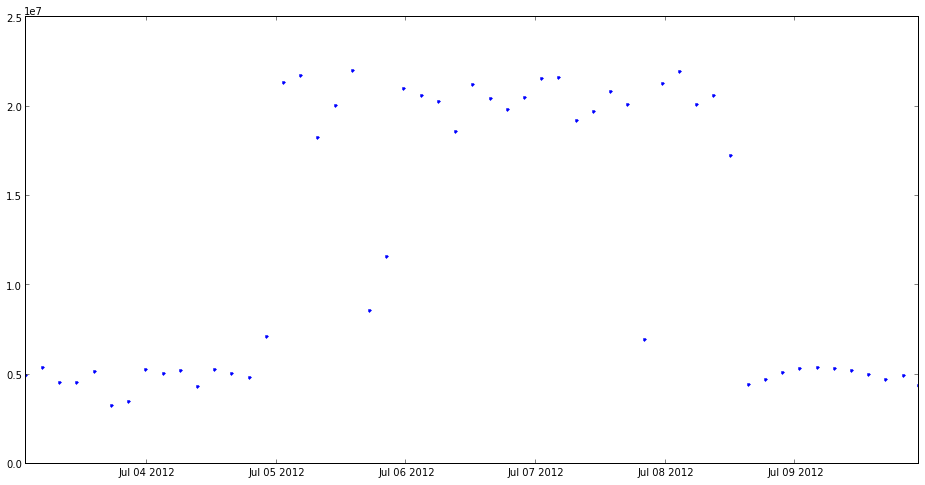Now we know what how the exposure on the crab changed for the 3 types of observations: regular survey, pointed and pointed/survey. It's clear that both pointed and pointed/survey worked equally well to increase the exposure on the Crab (and are about a factor of 4 better than survey mode).

All Sky Exposure maps for the 3 types of observation¶

In :


In :


In :
import os

In :
os.system("/Users/mcenery/software/ScienceTools/ScienceTools-v9r31p1-fssc-20130410-x86_64-apple-darwin12.2.0/x86_64-apple-darwin12.2.0/bin/gtltcube scfile=L1306040628475650A47F60_SC00.fits outfile=crab_ltcube_1.fits dcostheta=0.025 binsz=1.0 phibins=0 tmin=362966400.0 tmax=363052800.0 zmin=0.0 zmax=100.0 chatter=2 clobber=yes debug=no gui=no mode=ql evfile= ")

Out:
0
In :
os.system("/Users/mcenery/software/ScienceTools/ScienceTools-v9r31p1-fssc-20130410-x86_64-apple-darwin12.2.0/x86_64-apple-darwin12.2.0/bin/gtexpcube infile=crab_ltcube_1.fits cmfile=\"NONE\" outfile=expmap_crab_1_gal_10GeV.fits irfs=P7SOURCE_V6 nxpix=1 nypix=1 pixscale=1 coordsys=\"GAL\" xref=0 yref=0 axisrot=0 proj=\"AIT\" emin=10000 emax=10000 enumbins=1 bincalc=CENTER evfile=")

Out:
0
This makes an exposure map of the entire sky at 10 GeV for the first day (86400s) of the observation, which was regular survey mode. The output (written to the terminal) has the following as the last couple of lines Creating an Image, will write to file expmap_crab_1_gal_10GeV.fits Layer energy etendue miniumum mean maximum 0 10000 9489 6.24e+07 9.65e+07 1.79e+08 The mean exposure is a measure of the observation efficiency. If the LAT FoV is occulted by the Earth, then the zmax selection on ltcube will cause us to lose coverage on the sky. This will make the mean exposure drop. The minimum and maximum gives a sense of sky uniformity.
In :
os.system("/Users/mcenery/software/ScienceTools/ScienceTools-v9r31p1-fssc-20130410-x86_64-apple-darwin12.2.0/x86_64-apple-darwin12.2.0/bin/gtltcube scfile=L1306040628475650A47F60_SC00.fits outfile=crab_ltcube_2.fits dcostheta=0.025 binsz=1.0 phibins=0 tmin=363137731.0 tmax=363224131.0 zmin=0.0 zmax=100.0 chatter=2 clobber=yes debug=no gui=no mode=ql evfile= ")
os.system("/Users/mcenery/software/ScienceTools/ScienceTools-v9r31p1-fssc-20130410-x86_64-apple-darwin12.2.0/x86_64-apple-darwin12.2.0/bin/gtexpcube infile=crab_ltcube_2.fits cmfile=\"NONE\" outfile=expmap_crab_2_gal_10GeV.fits irfs=P7SOURCE_V6 nxpix=1 nypix=1 pixscale=1 coordsys=\"GAL\" xref=0 yref=0 axisrot=0 proj=\"AIT\" emin=10000 emax=10000 enumbins=1 bincalc=CENTER evfile=")

Out:
0
We then looked at a day of the purely pointed mode observation. We expect this to be much more uneven sky coverage. The last lines in the output for gtexpcube are: Creating an Image, will write to file expmap_crab_2_gal_10GeV.fits Layer energy etendue miniumum mean maximum 0 10000 9489 0 7.35e+07 2.77e+08 Now the minimum is 0, which means that at least one part of the sky got no exposure at all. The mean is 24% lower, which means that we are losing a significant amount of exposure due to occultation of the LAT FoV by the Earth. So this is good for the Crab (based on the exposure vs time plot that we made earlier) but terrible for the rest of the sky.
In :
os.system("/Users/mcenery/software/ScienceTools/ScienceTools-v9r31p1-fssc-20130410-x86_64-apple-darwin12.2.0/x86_64-apple-darwin12.2.0/bin/gtltcube scfile=L1306040628475650A47F60_SC00.fits outfile=crab_ltcube_3.fits dcostheta=0.025 binsz=1.0 phibins=0 tmin=363223719.0 tmax=363310119.0 zmin=0.0 zmax=100.0 chatter=2 clobber=yes debug=no gui=no mode=ql evfile= ")
os.system("/Users/mcenery/software/ScienceTools/ScienceTools-v9r31p1-fssc-20130410-x86_64-apple-darwin12.2.0/x86_64-apple-darwin12.2.0/bin/gtexpcube infile=crab_ltcube_3.fits cmfile=\"NONE\" outfile=expmap_crab_3_gal_10GeV.fits irfs=P7SOURCE_V6 nxpix=1 nypix=1 pixscale=1 coordsys=\"GAL\" xref=0 yref=0 axisrot=0 proj=\"AIT\" emin=10000 emax=10000 enumbins=1 bincalc=CENTER evfile=")

Out:
0
This is much better. For this day's observation we have recovered exposure over the entire sky. The mean exposure is 3% lower than the regular sky survey. Creating an Image, will write to file expmap_crab_3_gal_10GeV.fits Layer energy etendue miniumum mean maximum 0 10000 9489 1.46e+07 9.39e+07 3.12e+08Let's rescale the exposure maps by the mean of the survey mode case, so that we can more easily compare them with one another.
In :
os.system("farith expmap_crab_1_gal_10GeV.fits 9.65e+07  expmap_crab_1_gal_10GeV_scale.fits div NULL=yes")
os.system("farith expmap_crab_2_gal_10GeV.fits 9.65e+07  expmap_crab_2_gal_10GeV_scale.fits div NULL=yes")
os.system("farith expmap_crab_3_gal_10GeV.fits 9.65e+07  expmap_crab_3_gal_10GeV_scale.fits div NULL=yes")

Out:
26880
And now use ds9 to view them.
In :
Image(filename='crab_exposures.jpg')

Out: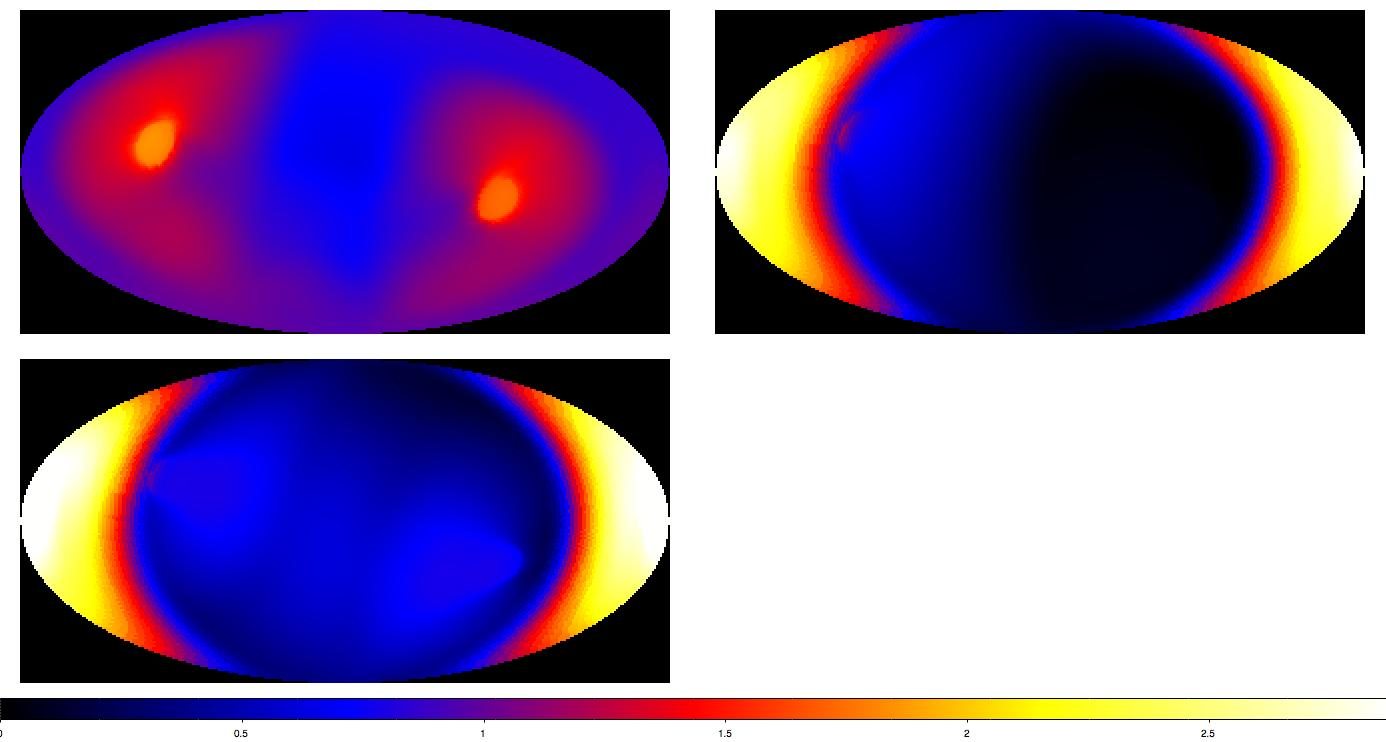In :
HTML("<iframe src='http://fermi.gsfc.nasa.gov/ssc/observations/timeline/ft2/' width='1000' height='500'></iframe>")

Out:
Once you have the spacecraft file, you need to create a dummy photon file if you want to be able to get exposure as a function of time at a specified spot on the sky. You can make one easily with gtobssim. I use the following input files (it really doesn't matter at all what source is used for this. src_sim.xml contains the following and source.dat has a single entry: _3C279
In :
os.system("/Users/mcenery/software/ScienceTools/ScienceTools-v9r31p1-fssc-20130410-x86_64-apple-darwin12.2.0/x86_64-apple-darwin12.2.0/bin/gtobssim infile=src_sim.xml srclist=source.dat scfile=FERMI_POINTING_FINAL_298_2014044_2014051_00.fits evroot=test simtime=150000 tstart=413942400. use_ac=no irfs=P6_V3_TRANSIENT seed=21341")

Out:
0
Now that I have a photon file (test_events_0000.fits) I can repeat the analysis from above.